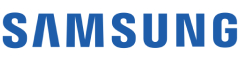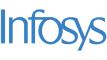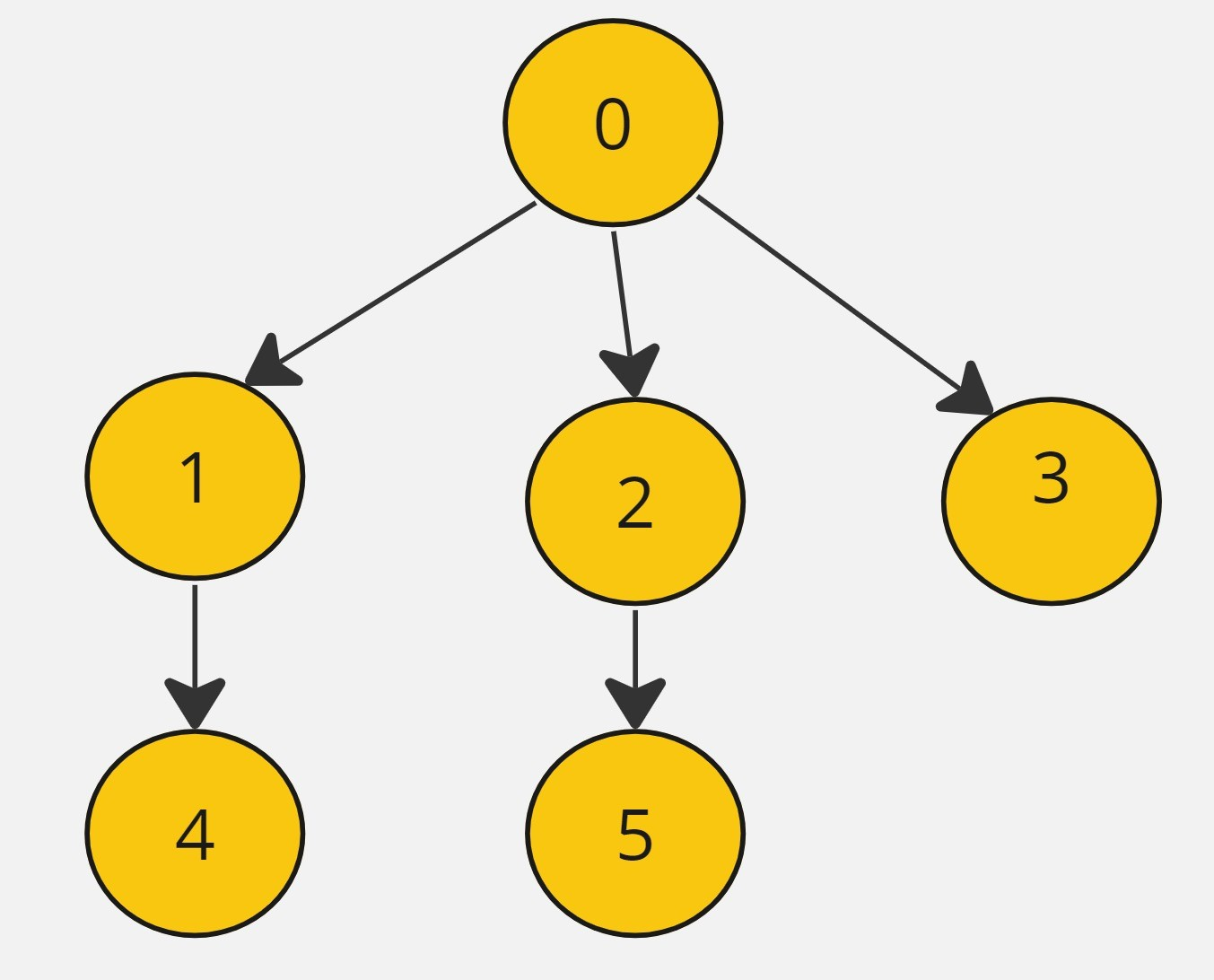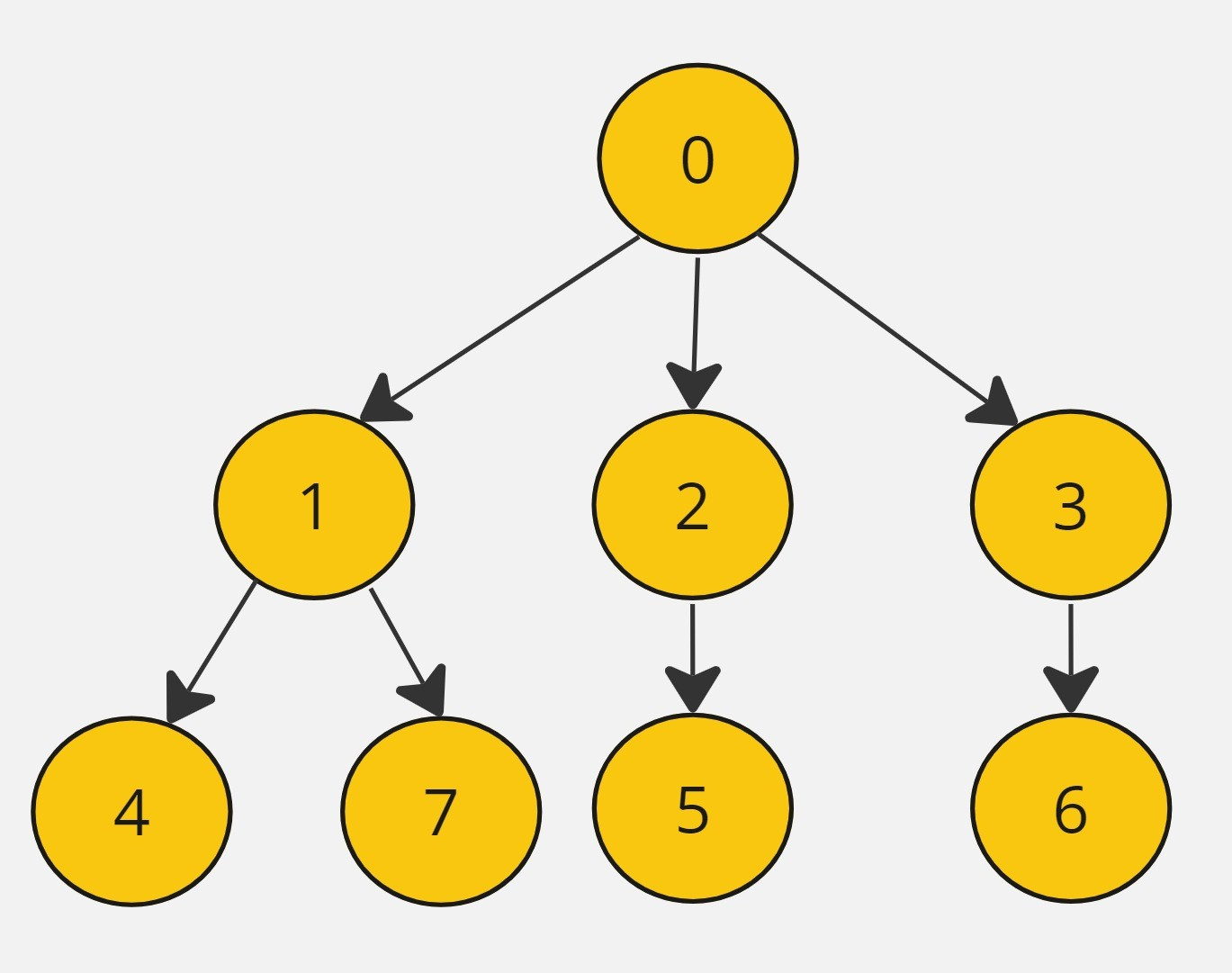New update is available. Click here to update.
Topics

# BFS in Graph

Easy0/40
Average time to solve is 10m+19 more companies

## Problem statement

Given an adjacency list representation of a directed graph with ‘n’ vertices and ‘m’ edges. Your task is to return a list consisting of Breadth-First Traversal (BFS) starting from vertex 0.

In this traversal, one can move from vertex 'u' to vertex 'v' only if there is an edge from 'u' to 'v'. The BFS traversal should include all nodes directly or indirectly connected to vertex 0.

Note:
``````The traversal should proceed from left to right according to the input adjacency list.
``````

Example:
``````Adjacency list: { {1,2,3},{4}, {5}, {},{},{}}

The interpretation of this adjacency list is as follows:
Vertex 0 has directed edges towards vertices 1, 2, and 3.
Vertex 1 has a directed edge towards vertex 4.
Vertex 2 has a directed edge towards vertex 5.
Vertices 3, 4, and 5 have no outgoing edges.

We can also see this in the diagram below.

BFS traversal: 0 1 2 3 4 5
``````Detailed explanation ( Input/output format, Notes, Images )
Sample Input 1:
``````8 7
0 1
0 2
0 3
1 4
1 7
2 5
3 6
``````
Sample Output 1:
``````0 1 2 3 4 7 5 6
``````
Explanation for sample input 1:``````n = 8: There are 8 vertices in the graph, labeled from 0 to 7.
m = 7: There are 7 directed edges in the graph.

The directed edges are as follows:
Vertex 0 has directed edges towards vertices 1, 2, and 3.
Vertex 1 has directed edges towards vertices 4 and 7.
Vertex 2 has a directed edge towards vertex 5.
Vertex 3 has a directed edge towards vertex 6.
Vertices 4, 5, 6, and 7 have no outgoing edges.

Adjacency list: {{1,2,3}, {4,7}, {5}, {6}, {}, {}, {}, {}}. This is passed to the bfsTraversal function.
``````
Sample Input 2:
``````4 4
0 1
0 2
1 2
0 3
``````
Sample Output 2:
``````0 1 2 3
``````
Constraints :
``````1 <= 'n', 'm' <= 10^4

Where 'n' is the number of vertices and 'm' is the number of edges.

Time Limit: 1 second
``````Console